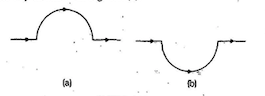Home/Class 12/Physics/

## QuestionPhysicsClass 12

A straight wire carrying a current of $$12\;A$$ is bent into a semi-circular arc of radius $$2.0\text{ cm}$$ as shown in Fig $$(a)$$. Consider the magnetic field $$B$$ at the Centre of the arc.
What is the magnetic field due to the straight segments?Fig.

$$\mathit{dl}$$  and $$r$$  for each element of the straight segments are parallel. Therefore, $$\mathit{dl}\times r=0$$ . Straight segment s do  not contribute to $$\mid B\mid$$.